Share

# Which of the following figures lie on the same base and between the same parallels. In such a case, write the common base and the two parallels. - CBSE Class 9 - Mathematics

ConceptFigures on the Same Base and Between the Same Parallels

#### Question

Which of the following figures lie on the same base and between the same parallels. In such a case, write the common base and the two parallels.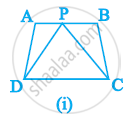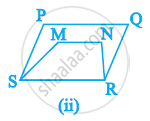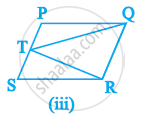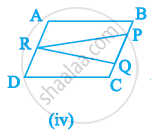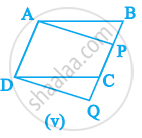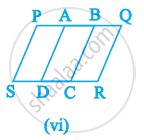#### SolutionYes. It can be observed that trapezium ABCD and triangle PCD have a common base CD and these are lying between the same parallel lines AB and CD.No. It can be observed that parallelogram PQRS and trapezium MNRS have a common base RS. However, their vertices, (i.e., opposite to the common base) P, Q of parallelogram and M, N of trapezium, are not lying on the same line.Yes. It can be observed that parallelogram PQRS and triangle TQR have a common base QR and they are lying between the same parallel lines PS and QR.No. It can be observed that parallelogram ABCD and triangle PQR are lying between same parallel lines AD and BC. However, these do not have any common base.Yes. It can be observed that parallelogram ABCD and parallelogram APQD have a common base AD and these are lying between the same parallel lines AD and BQ.No. It can be observed that parallelogram PBCS and PQRS are lying on the same base PS. However, these do not lie between the same parallel lines.

Is there an error in this question or solution?

#### APPEARS IN

RD Sharma Solution for Mathematics for Class 9 by R D Sharma (2018-19 Session) (2018 to Current)
Chapter 14: Areas of Parallelograms and Triangles
Ex. 14.10 | Q: 1 | Page no. 3
NCERT Solution for Mathematics Class 9 (2018 to Current)
Chapter 9: Areas of Parallelograms and Triangles
Ex. 9.10 | Q: 1 | Page no. 155

#### Video TutorialsVIEW ALL 

Solution Which of the following figures lie on the same base and between the same parallels. In such a case, write the common base and the two parallels. Concept: Figures on the Same Base and Between the Same Parallels.
S